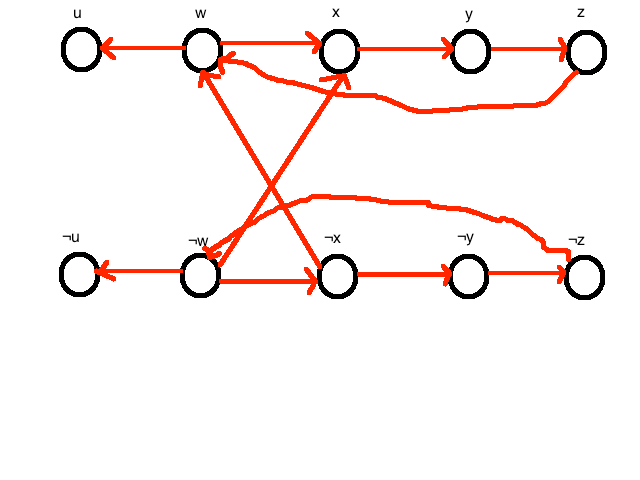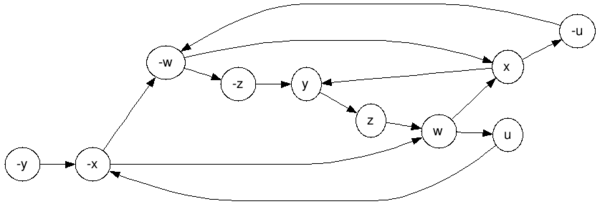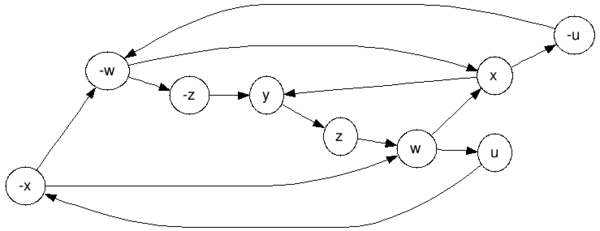# Drawing an implication graph for 2-SAT clausesProblem Detail: I am trying to convert the following 2-sat clauses to implications and then draw the implication graph. The clauses are: {¬xvy}, {¬yvz}, {¬zvw} ,{¬wvu},{¬uv¬x},{xvw},{¬wvx} I converted the boolean literals into implications so I could construct the implication graph: {¬xvy}: I have x–>y and ¬x –>¬y {¬yvz} : I have y–>z and ¬y–>¬z {¬zvw} : I have z–>w and ¬z–>¬w {¬wvu} : I have w–>u and ¬w—>¬u {¬uv¬x} : I have u–>¬x and ¬x–>¬u {xvw} : I have ¬x–>w and ¬w–>x {¬wvx} : I have w–>x and ¬w–>¬x Am I doing this right? If so, I have constructed this implication graph to prove it is not satisfiable.I would argue that these literals are not satisfiable because of the infinite loops you can have from ¬w ¬x ¬y ¬z ¬w and w x y z w. Is this a sufficient enough explanation? Thanks in advance!

#### Answered By : Realz Slaw

{¬uv¬x} : I have u–>¬x and ¬x–>¬u

LMFTFY: \$\$(neg u vee neg x) = left[(u implies neg x) wedge (ximplies neg u)right]\$\$

{¬wvx} : I have w–>x and ¬w–>¬x

LMFTFY: \$\$(neg w vee x) = left[(w implies x) wedge (neg ximplies neg w)right]\$\$ From this you get the following graph:graphviz source And the strongly connected components (there is only one):graphviz source And we get \$left{u,neg u,w,neg w, x,neg x,y,z,neg zright}\$, obviously a contradiction (the elements of this set implies each-other).

I would argue that these literals are not satisfiable because of the infinite loops you can have from ¬w ¬x ¬y ¬z ¬w and w x y z w. Is this a sufficient enough explanation?

It isn’t the “infinite” loops that make it unsatisfiable. “infinite loops” or cycles, make strongly connected components; and a 2sat formula is satisfiable iff (if and only if) there are no contradicting terms in any of its strongly connected components. In this graph there are clearly contradicting terms in the one large strongly connected component, as I demonstrated above, therefore it is unsatisfiable.